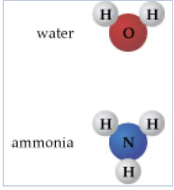Problem: Part C. A solution of ammonia and water contains 2.20×1025 water molecules and 8.80×1024 ammonia molecules. How many total hydrogen atoms are in this solution?Enter your answer numerically.Using mass measurements, early chemists were able to determine that for pure substances, the mass proportions of the composite elements were the same, no matter how much of the substance was analyzed. This became known as the law of definite proportions, or the law of constant composition. Dalton interpreted that this constant mass composition arose from constant composition of atoms. Working with this concept will help you to better understand the particulate nature of matter. To answer the questions, refer to the structures of water and ammonia shown here.

FREE Expert Solution
97% (480 ratings)Problem Details

Part C. A solution of ammonia and water contains 2.20×1025 water molecules and 8.80×1024 ammonia molecules. How many total hydrogen atoms are in this solution?

Using mass measurements, early chemists were able to determine that for pure substances, the mass proportions of the composite elements were the same, no matter how much of the substance was analyzed. This became known as the law of definite proportions, or the law of constant composition. Dalton interpreted that this constant mass composition arose from constant composition of atoms. Working with this concept will help you to better understand the particulate nature of matter. To answer the questions, refer to the structures of water and ammonia shown here.